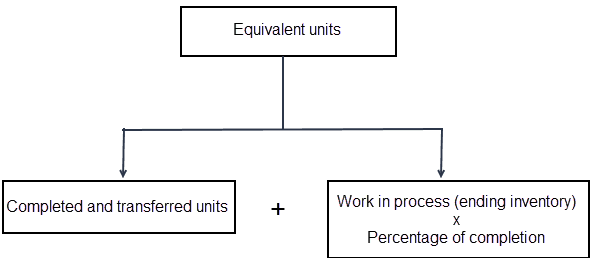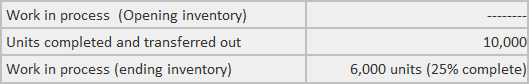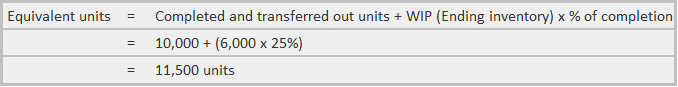## Equivalent Units of Production: Definition

The output of a department is always stated in terms of equivalent units of production.

The concept of equivalent units is defined as the number of units that would have been produced given the total amount of manufacturing effort expended for a given period.

## Explanation

The problem of work-in-progress in the process industries is a significant one.

In continuous processes, there is work-in-progress at the beginning and end of a period, as well as a degree of completion of closing work-in-progress.

Work-in-progress can be valued based on actual cost (i.e., an attempt may be made to find out how much materials have been used on the incomplete units and how much labor and expenses were used).

To solve the problem of work-in-progress, we can calculate equivalent units of production (or “effective production”). Equivalent or effective units of production represent the production of a process expressed in terms of completed units.

## Formula for Equivalent Units of Production

The formula below returns a value for equivalent units of production:

Equivalent units of production = Actual number of units in manufacturing process x Percentage of work completed

For example, the closing stock of 200 units in a process, with 60% complete in respect of materials, wages, and overheads, is equivalent to 120 units (i.e., 200 x 60%), which are 100% complete.

### Why Calculate Equivalent Units of Production?

The reason why is because the figure of completed units alone is not an accurate measure of a department’s output since some of the department’s efforts during a period are expended on units that are only partially complete.

To measure output accurately, these partially completed units must be considered in the output computation.

Mathematically, this is done by converting the partially completed units into fully completed units and then adjusting the output figure.

### Calculation of Equivalent Units of Production

Calculating equivalent units of production involves the following four steps:

#### Step 1

First, the equivalent production of opening work-in-progress should be determined by taking into account the degree of work to be performed in the current period.

For example, if the opening work-in-progress is 500 units, 40% complete in all respects, then the degree of work to be performed in the current period is 60%.

In this case, the equivalent production for opening work-in-progress in the period is 300 units (i.e., 500 x 60%).

#### Step 2

Secondly, the number of units introduced and completed in the current period should be calculated. This involves deducting the closing work-in-progress from the amount introduced in the process during the current period.

#### Step 3

Thirdly, the equivalent units of production for the closing work-in-progress should be determined by considering the number of units of closing work-in-progress and the level of completed work.

If the closing work-in-progress is 800 units, 70% complete in all respects, the equivalent units of production of closing work-in-progress is 560 units (i.e., 800 x 70%).

#### Step 4

Finally, the equivalent units of production calculated via the previous three steps should be aggregated to ascertain the total output in terms of equivalent units or equivalent production.

A unit is not transferred out unless it is completed. In this way, every transferred out unit is an equivalent unit.

The units that remain in the ending work-in-process inventory, however, are not complete. Therefore, to convert the work-in-process inventory into equivalent units, it is important to keep the percentage of completion in the calculation.### Example

Suppose that department A of a company reports the following information for January 2019:Required: What is the output in January for department A?

### Solution

To solve this problem, calculate the equivalent units of production. This is done as follows:## Evaluation of Equivalent Units of Production

The evaluation of equivalent production is usually made by preparing the following three statements:
1. Statement of Equivalent Production: This statement shows the number of equivalent completed units.
2. Statement of Cost per Equivalent Unit: This statement is prepared to compute the element-wise cost per equivalent unit. The cost of each element (i.e., material, labor, and overhead) is divided by the equivalent units of production of that element.
3. Statement Showing the Apportionment of Costs or Statement of Evaluation: This statement shows the apportionment of the total process cost to the finished output and work-in-progress of the process.

### Why is equal to units in completed status?

When a unit starts processing it is called as open. The unit cannot be considered complete until it gets into the finished goods or work-in-progress depending on the process flow. In this case, the units that are waiting in line for their turn are counted as open units.

### What are the limitations of equivalent units?

The limitation of equivalent units computation is that it does not take into account the number of units completed in any specific unit. For example, let's assume that a company manufactured 2000 motorcycles for this year and 30% of motorcycles were lost due to defects. If these defects are non recurring then such units should be excluded from equivalent production.

### What is the difference between equivalent Units of Production and equivalent production?

Equivalent Units of Production is a more accurate method to determine whether the proposed output of the process will be able to meet or exceed that budgeted for. It allows us to accurately calculate if we are meeting our production. In production, units completed in a period is equivalent to units that got into the finished goods or work-in-progress. So, they are equal.

### How is equivalent production calculated?

Equivalent units can be computed through any of the following methods: (i) constant overhead rate method, (ii) fixed cost per unit method and (iii) weighted average method. Which one would you use? Each of them will be discussed in upcoming lessons.

### How is equivalent production related to job order costing?

Equivalent units are the basic concept in job order costing. The equivalent production for each department is determined, which is later used to calculate the cost per unit of each job order by apportioning their total costs on basis of equivalent units.

True is a Certified Educator in Personal Finance (CEPF®), a member of the Society for Advancing Business Editing and Writing, contributes to his financial education site, Finance Strategists, and has spoken to various financial communities such as the CFA Institute, as well as university students like his Alma mater, Biola University, where he received a bachelor of science in business and data analytics.

To learn more about True, visit his personal website, view his author profile on Amazon, his interview on CBS, or check out his speaker profile on the CFA Institute website.